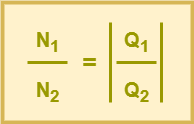# Electric Field-

Before you go through this article, make sure that you have gone through the previous article on Electric Field.

We have learnt-

• Electric field is the space around an electric charge in which any other charge experiences an electric force.
• Electric field intensity at a point is defined as the force experienced by a unit positive charge placed at that point.

## Electric Field Lines-

 Electric field lines are the pictorial representation of the electric field. OR Electric field line is an imaginary line such that the tangent at any point on the field line gives the direction of the electric field at that point.

## Properties of Electric Field Lines-

Some of the important properties of electric field lines are as follows-

### Property-01:

The electric field lines begin from a positive charge and terminate on a negative charge.

• For a positive point charge, electric field lines start from the positive charge and end at infinity.
• For a negative point charge, electric field lines start from infinity and end on the negative charge.### Property-02:

Electric field lines are the imaginary lines but the concept of electric field which they represent is real.

### Property-03:

The tangent at any point on an electric field line gives the direction of electric field at that point.

### Property-04:

Two electric field lines can never cross each other.

#### Reason

This is because if two electric field lines cross each other, then there will be two tangents at the point of intersection which means that there are two directions of the electric field at the point of intersection which is impossible.### Property-05:

Electric field lines do not form closed loops.

#### Reason

Electric field lines start from a positive charge and end on a negative charge.

They never go from a negative charge to a positive charge.

Hence, they do not form closed loops.

### Property-06:

Electric field lines are continuous curves without any break.

#### Reason

The electric field cannot have a sudden break because an electric charge experiences a continuous force in an electric field and does not jump from one point to another point in the field.

That is why, electric field lines are continuous curves.

### Property-07:

The relative closeness of electric field lines indicate the strength of electric field in that region.

The closer the lines are, the stronger is the electric field and vice-versa.

### Property-08:

Electric field lines contract longitudinally or lengthwise to represent attraction between two unlike charges.

### Property-09:

Electric field lines exert lateral (sideways) pressure on each other to represent repulsion between like charges.

### Property-10:

Electric field lines are always perpendicular to the surface of a charged sphere.

### Property-11:

Electric field lines do not pass through a conductor and the interior of a conductor is free from the influence of the electric field.

### Property-12:

The number of electric field lines coming out of a charge are directly proportional to the magnitude of the charge.

 If- N1 be the number of electric field lines due to a charge of magnitude Q1 N2 be the number of electric field lines due to a charge of magnitude Q2 Then-